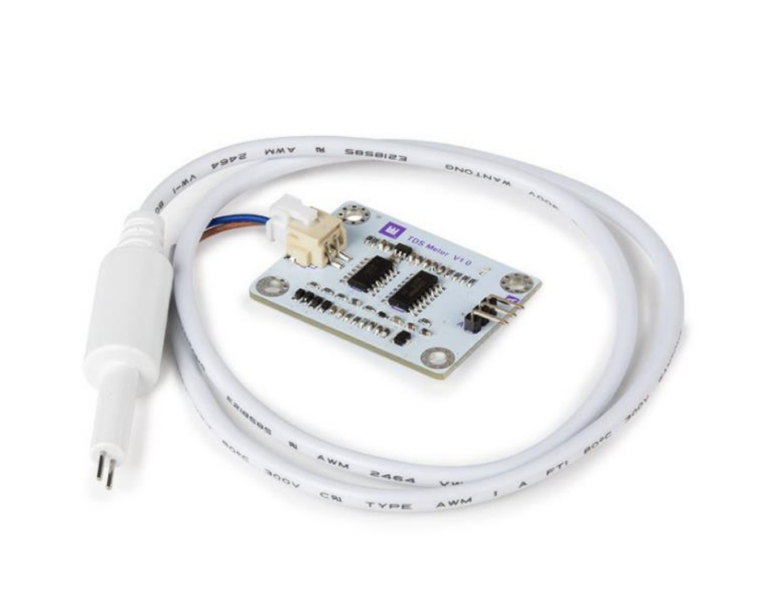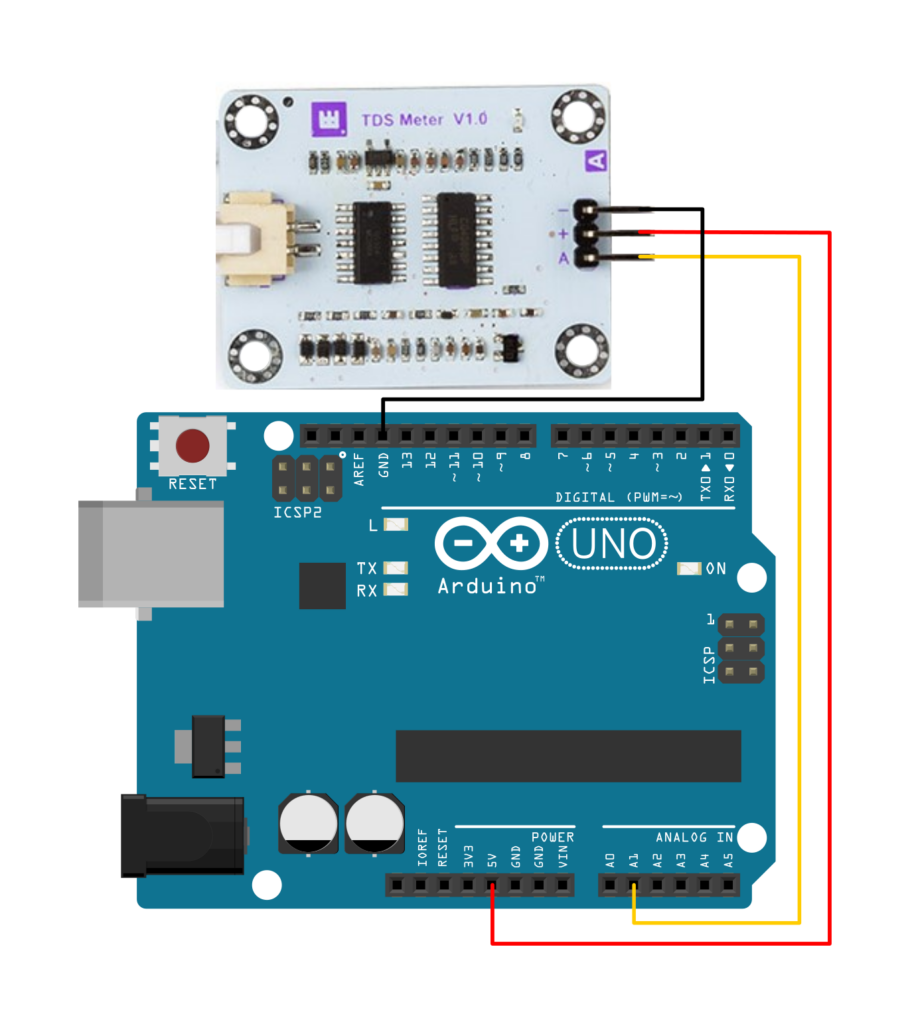# TDS Water Quality Sensor¶Use this sensitive measurement board to determine the quality of water based on its total dissolved solids or TDS. Find out whether your tap water is clean, or keep an eye on your aquarium water, and more!

This set includes the measurement board as well as a detachable water-proof measurement probe of 60 cm. The board has a standard 3-pin header interface connector and has a simple analog voltage readout.

The TDS measurement ranges from 0 – 1000 ppm, and has an accuracy of ± 10 % full-scale at 25 °C.

## Wiring Diagram¶

Pin Name Arduino® connection
`-` Ground GND
`+` Supply Voltage (3,3 - 5,5 V) 5V
`A` Analog sensor output signal Analog Pin (e.g. A1)## Example Code¶

 ``` 1 2 3 4 5 6 7 8 9 10 11 12 13 14 15 16 17 18 19 20 21 22 23 24 25 26 27 28 29 30 31 32 33 34 35 36 37 38 39 40 41 42 43 44 45 46 47 48 49 50 51 52 53 54 55 56 57 58 59 60 61 62 63 64 65 66``` ``````#define TdsSensorPin A1 // Define PIN number on Arduino #define VREF 5.0 // analog reference voltage(Volt) of the ADC #define SCOUNT 30 // sum of sample point int analogBuffer[SCOUNT]; // store the analog value in the array, read from ADC int analogBufferTemp[SCOUNT]; int analogBufferIndex = 0, copyIndex = 0; float averageVoltage = 0, tdsValue = 0, temperature = 25; void setup() { Serial.begin(9600); pinMode(TdsSensorPin, INPUT); // define seonsor Pin as input } void loop() { static unsigned long analogSampleTimepoint = millis(); if (millis() - analogSampleTimepoint > 40U) //every 40 milliseconds,read the analog value from the ADC { analogSampleTimepoint = millis(); analogBuffer[analogBufferIndex] = analogRead(TdsSensorPin); //read the analog value and store into the buffer analogBufferIndex++; if (analogBufferIndex == SCOUNT) analogBufferIndex = 0; } static unsigned long printTimepoint = millis(); if (millis() - printTimepoint > 800U) { printTimepoint = millis(); for (copyIndex = 0; copyIndex < SCOUNT; copyIndex++) analogBufferTemp[copyIndex] = analogBuffer[copyIndex]; averageVoltage = getMedianNum(analogBufferTemp, SCOUNT) * (float)VREF / 1024.0; // read the analog value more stable by the median filtering algorithm, and convert to voltage value float compensationCoefficient = 1.0 + 0.02 * (temperature - 25.0); //temperature compensation formula: fFinalResult(25^C) = fFinalResult(current)/(1.0+0.02*(fTP-25.0)); float compensationVolatge = averageVoltage / compensationCoefficient; //temperature compensation tdsValue = (133.42 * compensationVolatge * compensationVolatge * compensationVolatge - 255.86 * compensationVolatge * compensationVolatge + 857.39 * compensationVolatge) * 0.5; //convert voltage value to tds value //tdsValue is the variable used to store the ppm (parts per million) value. // Serial.print("voltage:"); //Serial.print(averageVoltage,2); //Serial.print("V "); Serial.print("TDS Value:"); //Serial ouput Serial.print(tdsValue, 0); //Serial ouput with value from sensor Serial.println("ppm"); //Serial ouput } } int getMedianNum(int bArray[], int iFilterLen) { int bTab[iFilterLen]; for (byte i = 0; i < iFilterLen; i++) bTab[i] = bArray[i]; int i, j, bTemp; for (j = 0; j < iFilterLen - 1; j++) { for (i = 0; i < iFilterLen - j - 1; i++) { if (bTab[i] > bTab[i + 1]) { bTemp = bTab[i]; bTab[i] = bTab[i + 1]; bTab[i + 1] = bTemp; } } } if ((iFilterLen & 1) > 0) bTemp = bTab[(iFilterLen - 1) / 2]; else bTemp = (bTab[iFilterLen / 2] + bTab[iFilterLen / 2 - 1]) / 2; return bTemp; } ``````